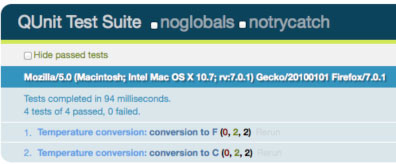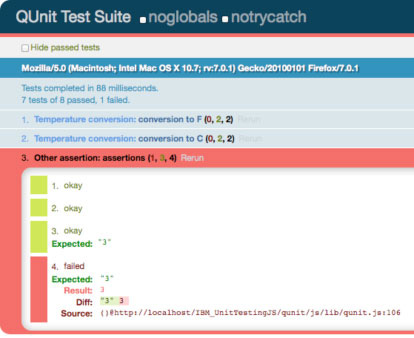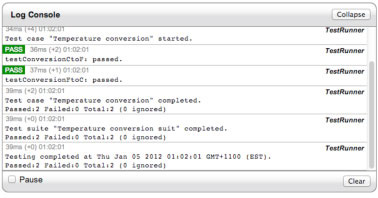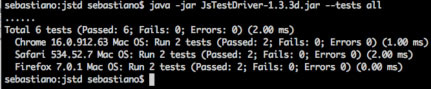求知 文章 文库 Lib 视频 iPerson 课程 认证 咨询 工具 讲座 Modeler Code分享到
JavaScript的单元测试工具

JavaScript单元测试

 ``` function addThreeToNumber(el){ return el + 3; } ```

 ``` (function testAddThreeToNumber (){ var a = 5, valueExpected= 8; if (addThreeToNumber (a) === valueExpected) { console.log("Passed!"); } else { console.log("Failed!"); } })(); ```

1. 在某个充当测试运行器的HTML页面中导入两个脚本文件，如清单3所示。

2. 在浏览器中打开该页面。

 ``` < !DOCTYPE html> < html> < head> < meta http-equiv="Content-type" content="text/html; charset=utf-8"> < title>Example 1< /title> < script type="text/javascript" src="js/script.js" kesrc="js/script.js">< /script> < script type="text/javascript" src="js/test.js" kesrc="js/test.js">< /script> < /head> < body>< /body> < /html> ```

QUnit入门

QUnit是一个类似于JUnit（Java编程）的单元测试框架，其被JQuery团队用来测试jQuery库。若要使用QUnit，你需要：

1. 下载qunit.css和qunit.js文件（参见参考资料）。

2. 创建一个HTML页面，该页面包含了一些导入你刚下载的那些CSS和JavsScript文件的特定标签。

 ``` < !DOCTYPE html> < html> < head> < meta charset="UTF-8" /> < title>QUnit Test Suite< /title> < link rel="stylesheet" href="css/qunit.css" kesrc="css/qunit.css" type="text/css" media="screen"> < script type="text/javascript" src="js/lib/qunit.js" kesrc="js/lib/qunit.js">< /script> < /head> < body> < h1 id="qunit-header">QUnit Test Suite< /h1> < h2 id="qunit-banner">< /h2> < div id="qunit-testrunner-toolbar">< /div> < h2 id="qunit-userAgent">< /h2> < ol id="qunit-tests">< /ol> < div id="qunit-fixture">test markup< /div> < /body> < /html> ```

 ``` function convertFromCelsiusToFahrenheit(c){ var f = c * (9/5) + 32; return f; } function convertFromFahrenheitToCelsius(f){ var c = (f - 32) * (5/9); return c; } ```

 ``` module ("Temperature conversion") test("conversion to F", function(){ var actual1 = convertFromCelsiusToFahrenheit(20); equal(actual1, 68, ?Value not correct?); var actual2 = convertFromCelsiusToFahrenheit(30); equal(actual2, 86, ?Value not correct?); }) test("conversion to C", function(){ var actual1 = convertFromFahrenheitToCelsius(68); equal(actual1, 20, ?Value not correct?); var actual2 = convertFromFahrenheitToCelsius(86); equal(actual2, 30, ?Value not correct?); }) ```

QUnit中的测试用例由test()方法来定义，逻辑包含在传递给该函数的第二个参数中。在清单6中，这两个测试被分别命名为conversion to F和conversion to C。每个测试都包含了两个断言，这些用在测试中的断言利用了equal()方法，equal()函数让你比较预期的值和被测试的函数返回的实际值。 equal()方法中的第三个参数是在失败情况下显示的信息。

1. 把源代码和测试文件放入到HTML测试器中，如清单7所示.

2. 在浏览器中打开该HTML页面。

 ``` ...``` module ("Other assertion"); test("assertions", function(){ ok(true); ok(3); strictEqual("c", "c"); equal (3, "3"); }); ```

ok()函数检查第一个参数为true；strictEqual()验证第一个参数与第二个严格相等。幕后实情是，strictEqual()使用了===运算符，而 equal()使用的是==运算符。

 ``` module ("Other assertion"); test("assertions", function(){ ok(true); ok(3); strictEqual("c", "c"); strictEqual (3, "3"); }); ```QUnit的另一个很重要的特性是，其允许你在某个模块中的所有测试被执行之前先、或之后再执行一些命令。module()函数把setup()和teardown()回调当成第二参数来接收，使用setup()函数来更新清单6，如清单10所示。

 ``` module ("Temperature conversion", { setup : function() { this.celsius1 = 20; this.celsius2 = 30; this.fahrenheit1 = 68; this.fahrenheit2 = 86; } }); test("conversion to F", function(){ var actual1 = convertFromCelsiusToFahrenheit(this.celsius1); equal(actual1, this.fahrenheit1); var actual2 = convertFromCelsiusToFahrenheit(this.celsius2); equal(actual2, this.fahrenheit2); }); test("conversion to C", function(){ var actual1 = convertFromFahrenheitToCelsius(this.fahrenheit1); equal(actual1, this.celsius1); var actual2 = convertFromFahrenheitToCelsius(this.fahrenheit2); equal(actual2, this.celsius2); }); ```

QUnit 还通过asyncTest()函数来提供异步测试支持，如果你正在使用Asynchronous JavaScript and XML（Ajax）来进行事务处理的话，则这是一个非常有用的功能。在这一上下文中，expect()函数能够让你轻松地验证运行在一个测试中的多个断言。

YUI Test：一个独立的单元测试模块

YUI Test，YUI库（Yahoo!）内部的一个组件，是一个详尽完整的单元测试框架。若要开始使用YUI Test，你需要：

1. 把YUI种子导入HTML运行器中，如下.

 ```

 ``` YUI().use("test", "console", function (Y) { // 测试用例放在这里 }); ```

test模块显然是出于测试目的必需的，console模块则不是强制要用的，不过该例子会使用它来输出结果。测试用例会放在回调的内部，使用一个全局的Y实例来作为参数。

 ``` YUI().use("test", "console", function (Y) { var suite = new Y.Test.Suite("Temperature conversion suite"); //add a test case suite.add(new Y.Test.Case({ name: "Temperature conversion? )); }); ```

 ``` suite.add(new Y.Test.Case({ name: "Temperature conversion", setUp : function () { this.celsius1 = 20; this.celsius2 = 30; this.fahrenheit1 = 68; this.fahrenheit2 = 86; }, testConversionCtoF: function () { Y.Assert.areEqual(this.fahrenheit1, convertFromCelsiusToFahrenheit(this.celsius1)); Y.Assert.areEqual(this.fahrenheit2, convertFromCelsiusToFahrenheit(this.celsius2)); }, testConversionFtoC: function () { Y.Assert.areEqual(this.celsius1, convertFromFahrenheitToCelsius(this.fahrenheit1)); Y.Assert.areEqual(this.celsius2, convertFromFahrenheitToCelsius(this.fahrenheit2)); } })); ```

1. setUp()方法是可用的。YUI在测试用例和测试套件层面都提供了setUp()和tearDown()方法。

2. 测试方法的名称以test这一词作为开始，他们包含有断言。

3. 该例子使用的是Y.Assert.areEqual()这一断言类型，其类似于QUnit中的equal()函数。

4. YUI Test提供了多种多样的断言方法，比如说：

4.1. Y.Assert.areSame()，该断言相当于QUnit中的strictEqual()。

4.2. 数据类型断言（Y.Assert.isArray(), Y.Assert.isBoolean(), Y.Assert.isNumber()等）。

4.3. 特殊值的断言（Y.Assert.isFalse(), Y.Assert.isNaN(), Y.Assert.isNull()等）。

 ``` Y.Test.Runner.add(suite); Y.Test.Runner.run(); ```

 ``` var console = new Y.Console({ verbose: true, newestOnTop: false, width: "600px" }); console.render('#testLogger'); ```

HTML运行器需要更新，添加控制台所引用的DOM元素，如清单16所示。

 ```
< /div> ```JSTestDriver带来的轻松测试

 ``` server: http://localhost:4224 load: - js/src/*.js test: - js/test/*.js ```

1. 启动测试服务器。通过命令行，进入到存放jsTestDriver.jar的文件夹，然后运行下面的命令：

1.java -jar JsTestDriver-1.3.3d.jar -port 4224

2. 复制和粘贴http://localhost:4224/capture这一URL到用于测试的浏览器中，以此来在服务器中注册一个或多个浏览器。

 ``` TestCase("Temperature conversion", { setUp : function () { this.celsius1 = 20; this.celsius2 = 30; this.fahrenheit1 = 68; this.fahrenheit2 = 86; }, testConversionCtoF: function () { assertSame(this.fahrenheit1, convertFromCelsiusToFahrenheit(this.celsius1)); assertSame(this.fahrenheit2, convertFromCelsiusToFahrenheit(this.celsius2)); }, testConversionFtoC: function () { assertSame(this.celsius1, convertFromFahrenheitToCelsius(this.fahrenheit1)); assertSame(this.celsius2, convertFromFahrenheitToCelsius(this.fahrenheit2)); } }); ```

java -jar JsTestDriver-1.3.3d.jar --tests allJSTD也可以很好地和你首选的持续集成系统整合在一起，成为持续构建的一部分。其提供了与诸如Eclipse（插件）或 TextMate（包）一类的IDE之间的集成。

1. QUnit，非常简单，是一个很好的入门框架。

2. YUI Test是一个完整的工具，适合熟悉YUI库的人。

3. JSTestDriver是一个非常不错的框架，可用来在多个浏览器中运行测试。

 LoadRunner性能测试基础 软件测试结果分析和质量报告 面向对象软件测试技术研究 设计测试用例的四条原则 功能测试中故障模型的建立 性能测试综述

 性能测试方法与技术 测试过程与团队管理 LoadRunner进行性能测试 WEB应用的软件测试 手机软件测试 白盒测试方法与技术

 某博彩行业 数据库自动化测试 IT服务商 Web安全测试 IT服务商 自动化测试框架 海航股份 单元测试、重构 测试需求分析与测试用例分析 互联网web测试方法与实践 基于Selenium的Web自动化测试